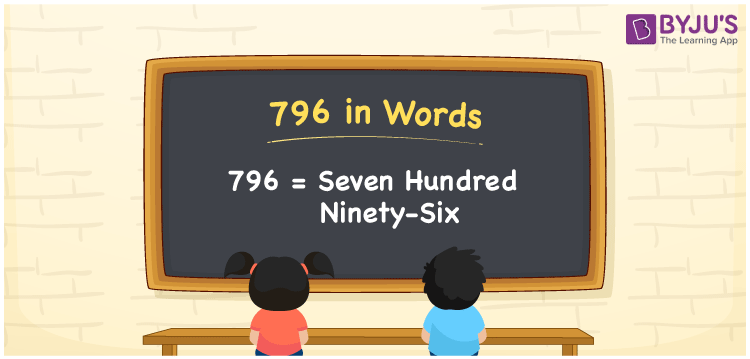# 796 in words

796 in words is written as Seven Hundred and Ninety Six. 796 represents the count or value. The article on Counting Numbers can give you an idea about count or counting. The number 796 is used in expressions that relate to money, distance, length, year and others. Let us consider an example for 796. ”Seven Hundred and Ninety Six days is more than 2 years”.

 796 in words Seven Hundred and Ninety Six Seven Hundred and Ninety Six in Numbers 796

## 796 in English Words## How to Write 796 in Words?

We can convert 796 to words using a place value chart. The number 796 has 3 digits, so let’s make a chart that shows the place value up to 3 digits.

 Hundreds Tens Ones 7 9 6

Thus, we can write the expanded form as:

= 7 × Hundred + 9 × Ten + 6 × One

= 7 × 100 + 9 × 10 + 6 × 1

= 796

= Seven Hundred and Ninety Six.

796 is the natural number that is succeeded by 795 and preceded by 797.

796 in words – Seven Hundred and Ninety Six.

Is 796 an odd number? – No.

Is 796 an even number? – Yes.

Is 796 a perfect square number? – No.

Is 796 a perfect cube number? – No.

Is 796 a prime number? – No.

Is 796 a composite number? – Yes.

## Solved Example

1. Write the number 796 in expanded form

Solution: 7 × 100 + 9 × 10 + 6 × 1

We can write 796 = 700 + 90 + 6

= 7 × 100 + 9 × 10 + 6 × 1

## Frequently Asked Questions on 796 in words

Q1

### How to write 796 in words?

796 in words is written as Seven Hundred and Ninety Six.
Q2

### Is 796 a perfect square number?

No. 796 is not a perfect square number.
Q3

### Is 796 a prime number?

No. 796 is not a prime number.